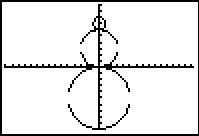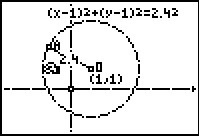# Activities

••• ##### Subject Area

• Math: Geometry: Circles

• ##### Author9-12

20 Minutes

• ##### Device
• TI-83 Plus Family
• TI-84 Plus
• TI-84 Plus Silver Edition
•TI-84 Plus C Silver Edition
•TI-84 Plus CE
• ##### Software

TI Connect™
TI Connect™ CE

• ##### Accessories

TI Connectivity Cable

## Exploring Circle Equations#### Activity Overview

Students explore the equation of a circle by connecting the coordinates of the center of the circle and the length of the radius to the corresponding parts of the equation.

#### Key Steps

•Students explore the equation of a circle. They will make the connection with the coordinates of the center of the circle and the length of the radius to the corresponding parts of the equation.

•Then, students apply what they have learned to find the equation of the circles in several circular designs.